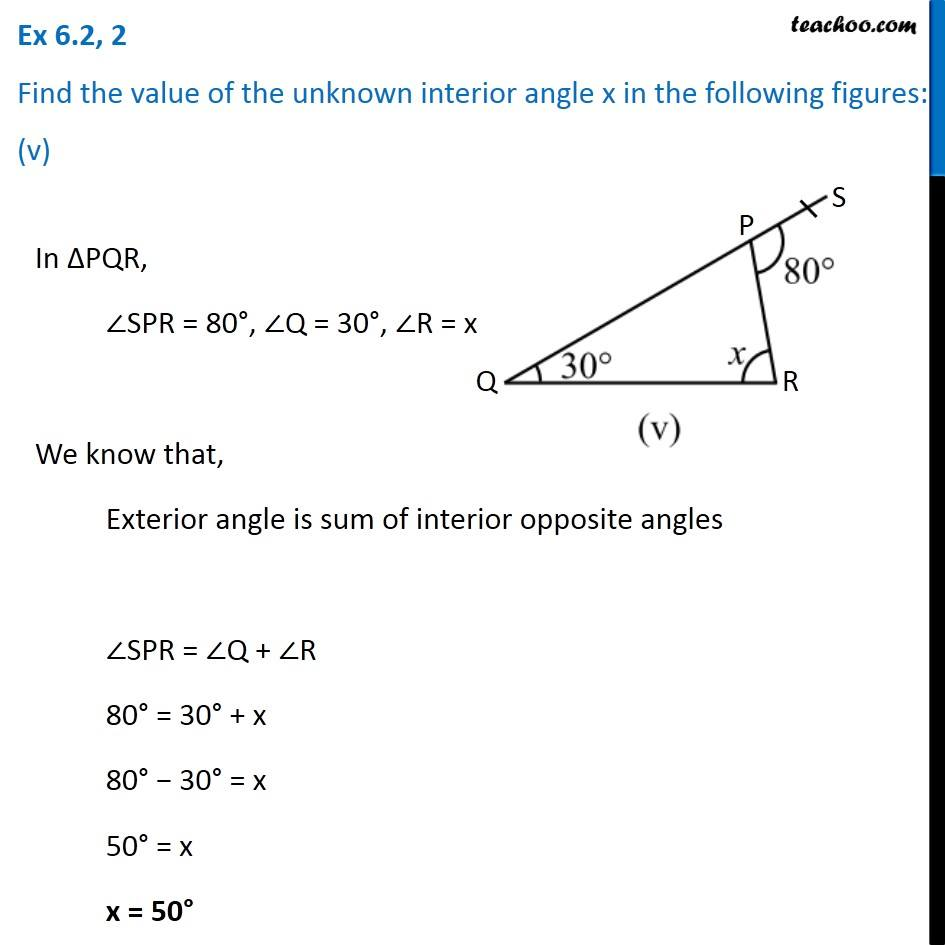Ex 6.2

Chapter 6 Class 7 Triangle and its Properties
Serial order wiseLearn in your speed, with individual attention - Teachoo Maths 1-on-1 Class

### Transcript

Ex 6.2, 2 Find the value of the unknown interior angle x in the following figures: (v)In ∆PQR, ∠SPR = 80°, ∠Q = 30°, ∠R = x We know that, Exterior angle is sum of interior opposite angles ∠SPR = ∠Q + ∠R 80° = 30° + x 80° − 30° = x 50° = x x = 50°